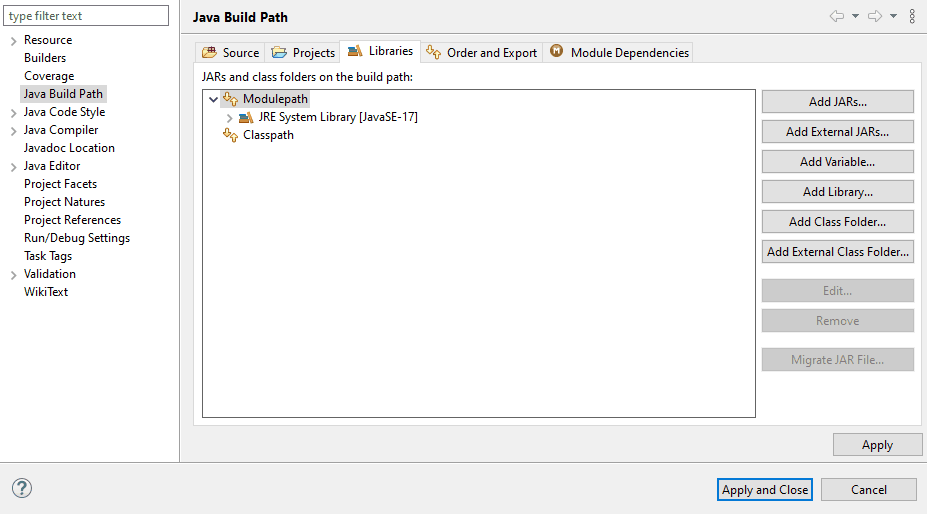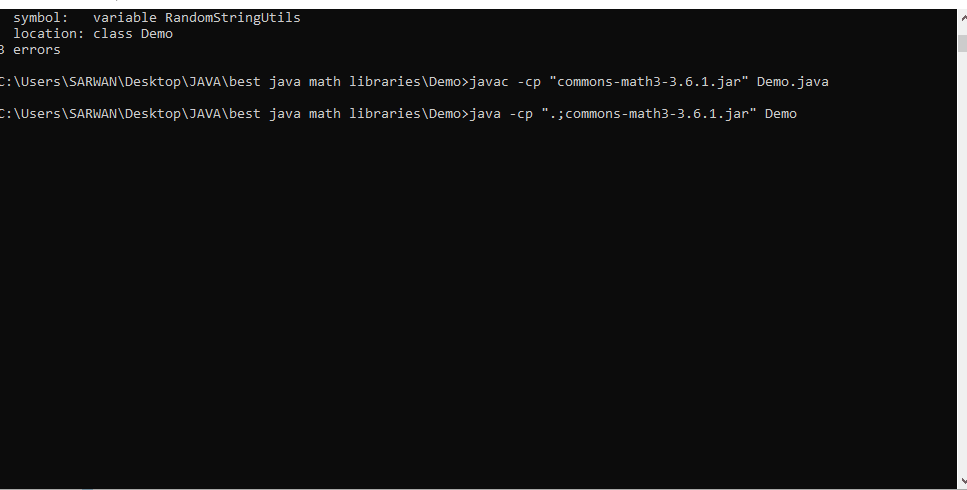# Best Math Libraries Every Java Developer Should Know

Sarwan Soomro Jan 03, 2023 Mar 20, 2022

Besides Java’s math library, there are powerful math libraries that you can extend to implement advanced math tasks in your Java projects.

To keep this tutorial precise, we will document the configuration of two well-known libraries for Java advanced math projects. And we are going to run two programs also using Apache Commons Math.

We will use CMD and IDE to implement and run our programs.

## Scope of Using Math Libraries in Java

Undoubtedly, most of our tasks can efficiently be concerned with Java’s math library. And most of us would not want to download `jar` files and add them as libraries to do something that could have been done using Java itself.

Well, not everyone is a Java nerd. Some are beginners.

Others switch technologies for a research problem statement, which can be as simple as rational numbers, or as complex as machine learning, deep learning, genetic algorithms, to name a few.

In both cases, using a library is not an issue. It will enable you to understand how developers build these classes to solve our problems.

Some credible Math Libraries a Java developer should know.

`Apache Commons` Apache Download Repository
`JScience` http://jscience.org/
`Parallel Colt` Resource Download
`Colt` Download
`Google Guava` Available
Note
There are tons of other libraries. We have included these based on their online popularity.

## Apache Commons Math Library in Java

It is a highly rated, trusted, and frequently used open-source math library from the famous Apache organization.

Check out some of its most popular functions:

1. Statistics
2. Data Generations
3. Linear Algebra
4. Numerical Analysis
5. Fractions
6. Geometry
7. Complex Numbers
8. Machine Learning
9. Optimization

Apache Common Math Libraries

Configuration:

Right-click your project from your favorite `IDE`, got to `build path` > `configure build path` > `libraries` > add external `jar` file.

Demo for Eclipse:## Configure Java Library From Windows Command Prompt

1. Create a folder `Demo`, paste your library `jar` file in the folder.

2. Now, create a simple program like the following (a demo for you, it can be anything that uses any library).

Code `Demo.java`:

``````import org.apache.commons.math3.fraction.Fraction;
public class Demo {

public static void main(String[] args) {
/*
* double[] n = { 1.1, 1.2, 1.3, 1.4, 2.1, 2.2, -2.35, 100.09 };
*
* for (double d : n) System.out.printf("12 : 445", d, new BigFraction(d,
* 0.00000002D, 10000));
*/

//In Java, assuming I have a double variable called decimal with the assigned value of 0.333,
//how would I display this as a "proper", formatted fraction -
//with the numerator over the denominator separated with a straight line (instead of simply displaying 1/3)?
Fraction a = new Fraction(1, 10);
Fraction b = new Fraction(0.99);
System.out.println("Result:" + " " + a.add(b));
// I need to add up a series of fractions, e.g. 1/2 + 1/3 + 1/4 + ..., and return a double. How can I avoid or minimize the round off error?
//
//        An alternative if you want to preserve accuracy,
//        and don't want to rely on the precision settings of BigDecimal, is to use the Apache Fractions library:

Fraction grf1 = Fraction.getReducedFraction(1, 2);
System.out.println(grf1);
System.out.println(grf1.doubleValue());
}
}
``````
3. Open Command Prompt in Windows.

4. Compile it: `javac -cp "commons-math3-3.6.1.jar" Demo.java`.

5. Run it: `java -cp ".;commons-math3-3.6.1.jar" Demo`.

Output:

``````Result: 109 / 100
4 / 3
1.3333333333333333
``````

If you have any confusion, we have created a demo gif too.## Apache Commons Demo Math Programs

The following code block will create random numbers and strings in the range as defined. You can use Apache Commons to play with alphabetic and numerics to create your algorithms.

It uses: `apache import org.apache.commons.lang3.RandomStringUtils;` of `commons-lang3-3.12.0` `jar` file (Also attached in the zip folder).

Code:

``````/*Basic level random string and random numeric value generator in the given range.abstract
Note this is for the demonstration of how to use a particular library*/
import org.apache.commons.lang3.RandomStringUtils;

public class BestJavaMathLibrary {
public static void main(String[] args) {

String rn1 = RandomStringUtils.randomNumeric(50);
System.out.println(rn1);
String rs1 = RandomStringUtils.randomAlphabetic(50);

System.out.println(rs1);
}

}
``````

Output:

``````80511636875416144724783964293309510956685562561281
``````

## JScience Library for Java

It is another credible math library for some Java advanced-level tasks. This library provides excellent support for rational numbers, polynomial rational, and vector rational.

You can also construct entries and extend their classes by applying your custom algorithm in Java, especially if you are a research student.

2. Configuration: You can follow the steps described in the previous section of this tutorial.

Demo Example:

Suppose you are going to do something related to rational numbers, and you want to use JScience class for that.

Code:

``````import java.util.Collection;

import org.jscience.mathematics.number.LargeInteger;
import org.jscience.mathematics.number.Rational;

public class JScienceDemo {
public static void main (String[] args) {